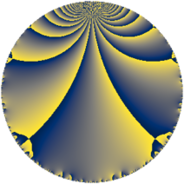# Properties

 Label 63.4.oLevel $63$ Weight $4$ Character orbit 63.o Rep. character $\chi_{63}(20,\cdot)$ Character field $\Q(\zeta_{6})$ Dimension $44$ Newform subspaces $1$ Sturm bound $32$ Trace bound $0$

# Related objects

## Defining parameters

 Level: $$N$$ $$=$$ $$63 = 3^{2} \cdot 7$$ Weight: $$k$$ $$=$$ $$4$$ Character orbit: $$[\chi]$$ $$=$$ 63.o (of order $$6$$ and degree $$2$$) Character conductor: $$\operatorname{cond}(\chi)$$ $$=$$ $$63$$ Character field: $$\Q(\zeta_{6})$$ Newform subspaces: $$1$$ Sturm bound: $$32$$ Trace bound: $$0$$

## Dimensions

The following table gives the dimensions of various subspaces of $$M_{4}(63, [\chi])$$.

Total New Old
Modular forms 52 52 0
Cusp forms 44 44 0
Eisenstein series 8 8 0

## Trace form

 $$44q - 6q^{2} + 78q^{4} + 5q^{7} + O(q^{10})$$ $$44q - 6q^{2} + 78q^{4} + 5q^{7} - 18q^{11} + 204q^{14} + 66q^{15} - 242q^{16} - 210q^{18} - 57q^{21} - 34q^{22} - 102q^{23} - 352q^{25} + 300q^{28} + 246q^{29} - 144q^{30} + 1068q^{32} - 1818q^{36} + 328q^{37} - 798q^{39} - 1158q^{42} - 170q^{43} + 968q^{46} - 79q^{49} + 288q^{50} - 204q^{51} + 1212q^{56} + 324q^{57} - 538q^{58} + 5316q^{60} + 783q^{63} - 808q^{64} + 4350q^{65} - 590q^{67} + 384q^{70} + 4284q^{72} - 5304q^{74} - 2787q^{77} + 612q^{78} - 302q^{79} - 3720q^{81} + 7050q^{84} - 612q^{85} - 13692q^{86} + 1294q^{88} + 210q^{91} - 10194q^{92} - 4266q^{93} + 6336q^{95} + 1014q^{99} + O(q^{100})$$

## Decomposition of $$S_{4}^{\mathrm{new}}(63, [\chi])$$ into newform subspaces

Label Dim. $$A$$ Field CM Traces $q$-expansion
$$a_2$$ $$a_3$$ $$a_5$$ $$a_7$$
63.4.o.a $$44$$ $$3.717$$ None $$-6$$ $$0$$ $$0$$ $$5$$